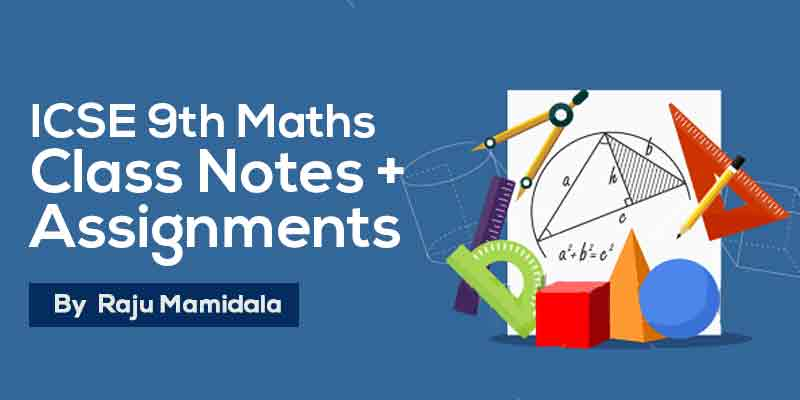## by Raju Mamidala

49 Users Enrolled
Class 9 , Maths
Board: ICSE , Language: English

Mode- Online
Contains- 24 PDFS
Access Duration- Till 30 Apr 2019

## WHAT WILL I LEARN?

We aim to prepare Class 9 ICSE students with a complete study material so that the students can score better in the school exam.

Simple and easy to understand.

Understand concepts better by these quick revision notes.

Student can test themself with assignments which are provided here.

## Course Description

ICSE Class 9 is an important stage where students need to concentrate on this subject as it is considered to be the groundwork of higher classes. To become clear with your aspiration and knowledge, you need to begin your preparation from this level. so here you can get complete notes and assignments for preparation along with solutions/Answers.

This study material will help you to better understand the topics and  these notes, students will be able to make quick revisions of topics and score good marks in there exams.

## Curriculum

Co ordinate geometry

Commercial mathematics

• Commercial mathematics - Class Notes
• Commercial mathematics - Work Assignment

Expansion and factorization

• Expansion and factorization - Class Notes
• Expansion and factorization - Work Assignment

Linear equations and simultaneous equations

• Linear equations and simultaneous equations - Class Notes
• Linear equations and simultaneous equations - Work Assignment

Logarithms and indices

• Logarithms and indices - Class Notes
• Logarithms and indices - Work Assignment

Perimeter and area of plane figures

• Perimeter and area of plane figures - Class Notes
• Perimeter and area of plane figures - Work Assignment

• Quadrilateral and polygons - Class Notes
• Quadrilateral and polygons - Work Assignment

Rational and irrational numbers

• Rational and irrational numbers - Class Notes
• Rational and irrational numbers - Work Assignment

Statistics

• Statistics - Class Notes
• Statistics - Work Assignment

Trigonometry

• Trigonometry - Class Notes
• Trigonometry - Work Assignment

volume and surface area of solids

• volume and surface area of solids - Class Notes
• volume and surface area of solids - Work Assignment

Triangles

• Triangles - Class Notes
• Triangles - Work Assignment

## About Raju Mamidala### Raju Mamidala

Mathematics | Class 6th to Competitive exams | 15+ Years Experience | Working in Geethanjali International School in Hyderabad from past 5 years. He Created an Educational Channel for improvement in various aspects for students in IIT Foundation.

#### If you have any doubts about how to get and access this course,watch this video.## 500 299

Includes 18 % GST

Mode- Online

Contains- 24 PDFS

Access Duration- Till 30 Apr 2019